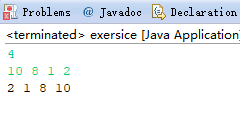# 崔志伟博客# Java版 将数组中的数逆序存放

### 输入样例:

4
10 8 1 2


### 输出样例:

2 1 8 10

import java.util.Scanner;
public class exersice {
public static void main(String[] args) {
Scanner in=new Scanner(System.in);
int N= in.nextInt();
int a[]=new int[N];
for(int i=0;i<a.length;i++){
a[i]=in.nextInt();
}
int temp;
for(int i=0;i<a.length/2;i++){
temp=a[i];
a[i]=a[a.length-i-1];
a[a.length-i-1]=temp;
}
for(int i=0;i<a.length;i++){
System.out.println(a[i]+" ");
}
}

}a,a交换

a,a交换